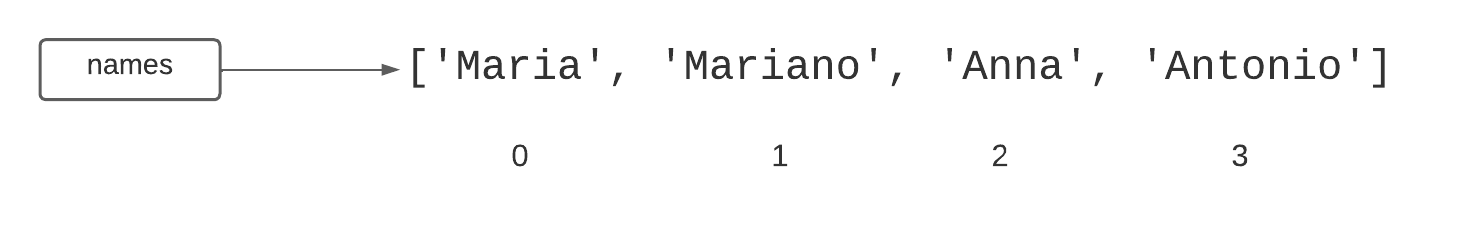Computer Science

# Lists

A list contains a collection of values. You have already seen variables that hold a single value:

number = 5

A variable that references a list can hold multiple values, in order:

numbers = [5, 8, 10, 13, 42]
names = ['Maria', 'Mariano', 'Anna', 'Antonino']

A list can hold as many values as you want. We can even have an empty list:

twilight_books_i_like = []

## Length

To get the length of a list, use len:

number_of_names = len(names)

Here, number_of_names will be equal to 4.

## Indexing

The items in a list are indexed starting from zero:To access individual items in the list, use square brackets:

grandmother = names

In this case, grandmother would have the value Anna. Likewise, names would be Maria.

We can count backwards from the end using negative numbers, so names[-1] is Antonino.

## Iteration

Once you have a list, you can iterate (or loop) through it using for ... in:

for number in numbers:
print(number)

Each time through the for loop, Python sets the variable number to one of the numbers in the list, in order. Since we have set the numbers variable to [5, 8, 10, 13, 42], the first time through the loop, number = 5. The second time through the loop, number = 8, and so forth. So this code will print:

5
8
10
13
42

The name of the variable can be whatever you like. For example:

for cute_puppies in numbers:
print(cute_puppies)

However, you want to be sure that your friends can read your code and make sense of it!

Your code is much more readable if you use variables that make sense.

## Append

One of the many functions we can perform on a list is appending items to it. This means adding items to the end of the list. For example:

pets = ['cat', 'horse', 'dog']
pets.append('emu')

This will result in the pets list now containing ['cat', 'horse', 'dog', 'emu'].